# Ex.6.3 Q5 Squares and Square Roots - NCERT Maths Class 8

## Question

For each of the following numbers, find the smallest whole number by which it should be multiplied so as to get a perfect square number. Also find the square root of the square number so obtained.

(i) $$252$$

(ii) $$180$$

(iii) $$1008$$

(iv) $$2028$$

(v) $$1458$$

(vi) $$768$$

Video Solution
Squares And Square Roots
Ex 6.3 | Question 5

## Text Solution

What is known?

Numbers

What is unknown?

The smallest whole number by which it should be multiplied so as to get a perfect square number

Reasoning:

To get a perfect square, each factor of the given number must be paired.

Steps:

(i) $$252$$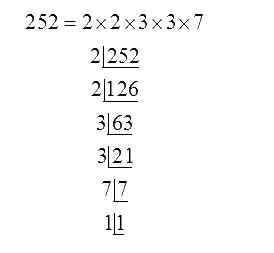Hence, Prime factor $$7$$ does not have its pair. If $$7$$ gets a pair, then the number becomes a perfect square. Therefore, $$252$$ has to be multiplied with $$7$$ to get a perfect square.

So, perfect square is $$252 \times 7 = 1764$$

$$1764 \! = \! 2 \! \times \! 2 \! \times \! 3 \! \times \! 3 \! \times \! 7 \! \times \! 7$$

So, perfect root of $$1764$$ is $$2 \times 3 \times 7 = 42$$

(ii) $$180$$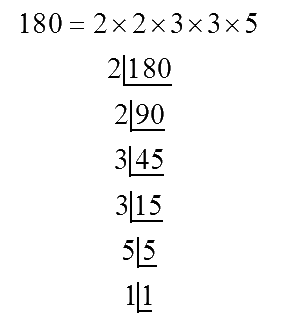Hence, prime factor $$5$$ does not have its pair. If $$5$$ gets a pair, then the number becomes a perfect square. Therefore, $$180$$ has to be multiplied with $$5$$ to get a perfect square.

So, perfect square is $$180 \times 5 = 900$$

$$900 \! = \! 2 \! \times \! 2 \! \times \! 3 \! \times \! 3 \! \times \! 5 \! \times \! 5$$

So, perfect root of $$900$$ is $$2 \times 3 \times 5 = 30$$

(iii) $$1008$$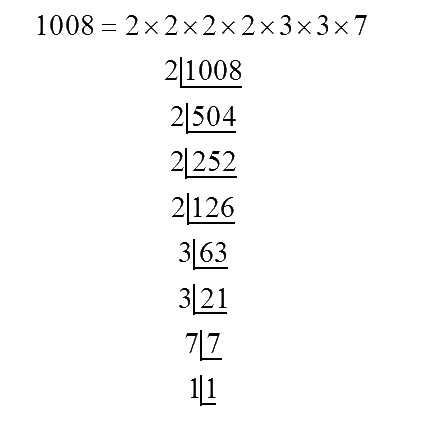Hence, prime factor $$7$$ does not have its pair. If $$7$$ gets a pair, then the number becomes a perfect square. Therefore, $$1008$$ has to be multiplied with $$7$$ to get a perfect square.

So, perfect square is $$1008 \times 7 = 7056$$

$$7056 \! = \! 2 \! \times \! 2 \! \times \! 2 \! \times \! 2 \! \times \! 3 \! \times \! 3 \! \times \! 7 \! \times \! 7$$

So, perfect root of

$$7056$$ is $$2 \times 2 \times 3 \times 7= 84$$

(iv) $$2028$$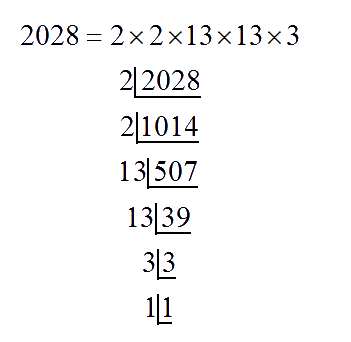Hence, prime factor $$3$$ does not have its pair. If $$3$$ gets a pair, then the number becomes a perfect square. Therefore, $$2028$$ has to be multiplied with $$3$$ to get a perfect square.

So, perfect square is $$2028 \times 3 = 6084$$

$$6084 \! = \! 2 \! \times \! 2 \! \times \! 13 \! \times \! 13 \! \times \! 3 \! \times \! 3$$

So, perfect root of $$6084$$ is $$2 \times 13 \times 3 = 78$$

(v) $$1458$$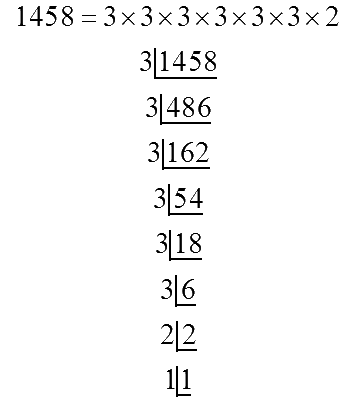Hence, prime factor $$2$$ does not have its pair. If $$2$$ gets a pair, then the number becomes a perfect square. Therefore, $$1458$$ has to be multiplied with $$2$$ to get a perfect square.

So, perfect square is $$1458 \times 2 = 2916$$

$$2916 \! = \! 3 \! \times \! 3 \! \times \! 3 \! \times \! 3 \! \times \! 3 \! \times \! 3 \! \times \! 2 \! \times \! 2$$

So, perfect root of $$2916$$ is $$3 \! \times \! 3 \! \times \! 3 \! \times \! 2 \! = \! 54$$

(vi) $$768$$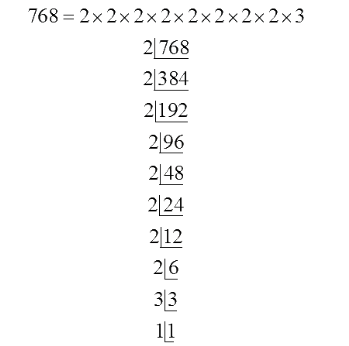Hence, prime factor $$3$$ does not have its pair. If $$3$$ gets a pair, then the number becomes a perfect square. Therefore, $$768$$ has to be multiplied with $$3$$ to get a perfect square.

So, perfect square is $$768 \times 3 = 2304$$

$$2304 \! = \! 2 \! \times \! 2 \! \times \! 2 \! \times \! 2 \! \times \! 2 \! \times \! 2 \! \times \! 2 \! \times \! 2 \! \times \! 3 \! \times \! 3$$

So, perfect root of $$2304$$ is $$2 \! \times \! 2 \! \times \! 2 \! \times \! 2 \! \times \! 3 \! = \! 48$$

Learn from the best math teachers and top your exams

• Live one on one classroom and doubt clearing
• Practice worksheets in and after class for conceptual clarity
• Personalized curriculum to keep up with school